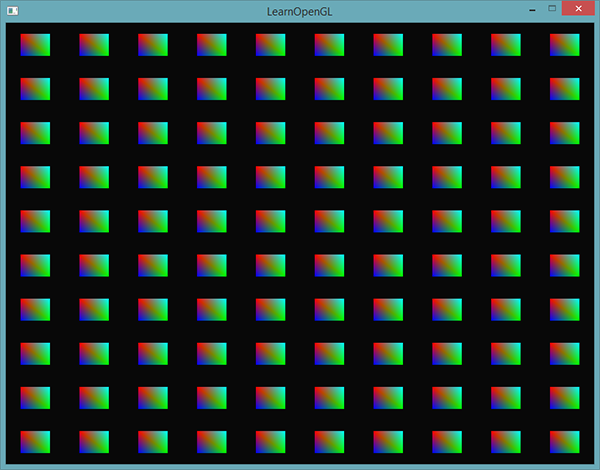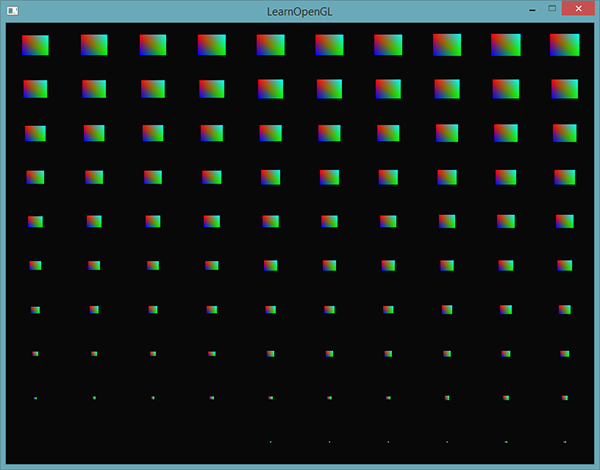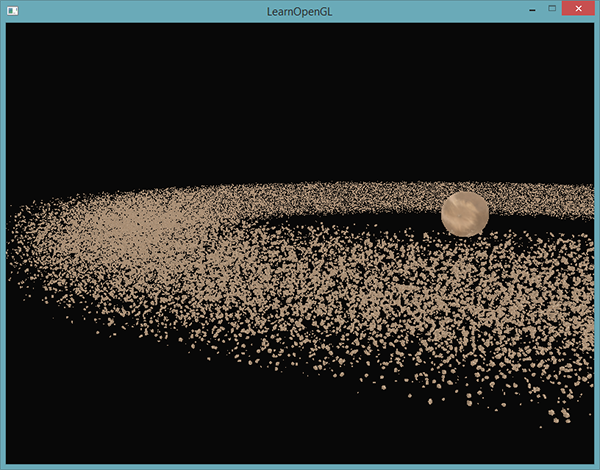# 实例化

for(unsigned int i = 0; i < amount_of_models_to_draw; i++)
{
DoSomePreparations(); // 绑定VAO，绑定纹理，设置uniform等
glDrawArrays(GL_TRIANGLES, 0, amount_of_vertices);
}float quadVertices[] = {
// 位置          // 颜色
-0.05f,  0.05f,  1.0f, 0.0f, 0.0f,
0.05f, -0.05f,  0.0f, 1.0f, 0.0f,
-0.05f, -0.05f,  0.0f, 0.0f, 1.0f,

-0.05f,  0.05f,  1.0f, 0.0f, 0.0f,
0.05f, -0.05f,  0.0f, 1.0f, 0.0f,
0.05f,  0.05f,  0.0f, 1.0f, 1.0f
};


#version 330 core
out vec4 FragColor;

in vec3 fColor;

void main()
{
FragColor = vec4(fColor, 1.0);
}


#version 330 core
layout (location = 0) in vec2 aPos;
layout (location = 1) in vec3 aColor;

out vec3 fColor;

uniform vec2 offsets;

void main()
{
vec2 offset = offsets[gl_InstanceID];
gl_Position = vec4(aPos + offset, 0.0, 1.0);
fColor = aColor;
}


glm::vec2 translations;
int index = 0;
float offset = 0.1f;
for(int y = -10; y < 10; y += 2)
{
for(int x = -10; x < 10; x += 2)
{
glm::vec2 translation;
translation.x = (float)x / 10.0f + offset;
translation.y = (float)y / 10.0f + offset;
translations[index++] = translation;
}
}


shader.use();
for(unsigned int i = 0; i < 100; i++)
{
stringstream ss;
string index;
ss << i;
index = ss.str();
shader.setVec2(("offsets[" + index + "]").c_str(), translations[i]);
}


glBindVertexArray(quadVAO);
glDrawArraysInstanced(GL_TRIANGLES, 0, 6, 100);


glDrawArraysInstanced的参数和glDrawArrays完全一样，除了最后多了个参数用来设置需要绘制的实例数量。因为我们想要在10x10网格中显示100个四边形，我们将它设置为100.运行代码之后，你应该能得到熟悉的100个五彩的四边形。

## 实例化数组

#version 330 core
layout (location = 0) in vec2 aPos;
layout (location = 1) in vec3 aColor;
layout (location = 2) in vec2 aOffset;

out vec3 fColor;

void main()
{
gl_Position = vec4(aPos + aOffset, 0.0, 1.0);
fColor = aColor;
}


unsigned int instanceVBO;
glGenBuffers(1, &instanceVBO);
glBindBuffer(GL_ARRAY_BUFFER, instanceVBO);
glBufferData(GL_ARRAY_BUFFER, sizeof(glm::vec2) * 100, &translations, GL_STATIC_DRAW);
glBindBuffer(GL_ARRAY_BUFFER, 0);


glEnableVertexAttribArray(2);
glBindBuffer(GL_ARRAY_BUFFER, instanceVBO);
glVertexAttribPointer(2, 2, GL_FLOAT, GL_FALSE, 2 * sizeof(float), (void*)0);
glBindBuffer(GL_ARRAY_BUFFER, 0);
glVertexAttribDivisor(2, 1);void main()
{
vec2 pos = aPos * (gl_InstanceID / 100.0);
gl_Position = vec4(pos + aOffset, 0.0, 1.0);
fColor = aColor;
}# 小行星带

unsigned int amount = 1000;
glm::mat4 *modelMatrices;
modelMatrices = new glm::mat4[amount];
srand(glfwGetTime()); // 初始化随机种子
float offset = 2.5f;
for(unsigned int i = 0; i < amount; i++)
{
glm::mat4 model;
// 1. 位移：分布在半径为 'radius' 的圆形上，偏移的范围是 [-offset, offset]
float angle = (float)i / (float)amount * 360.0f;
float displacement = (rand() % (int)(2 * offset * 100)) / 100.0f - offset;
float x = sin(angle) * radius + displacement;
displacement = (rand() % (int)(2 * offset * 100)) / 100.0f - offset;
float y = displacement * 0.4f; // 让行星带的高度比x和z的宽度要小
displacement = (rand() % (int)(2 * offset * 100)) / 100.0f - offset;
float z = cos(angle) * radius + displacement;
model = glm::translate(model, glm::vec3(x, y, z));

// 2. 缩放：在 0.05 和 0.25f 之间缩放
float scale = (rand() % 20) / 100.0f + 0.05;
model = glm::scale(model, glm::vec3(scale));

// 3. 旋转：绕着一个（半）随机选择的旋转轴向量进行随机的旋转
float rotAngle = (rand() % 360);
model = glm::rotate(model, rotAngle, glm::vec3(0.4f, 0.6f, 0.8f));

// 4. 添加到矩阵的数组中
modelMatrices[i] = model;
}


// 绘制行星
glm::mat4 model;
model = glm::translate(model, glm::vec3(0.0f, -3.0f, 0.0f));
model = glm::scale(model, glm::vec3(4.0f, 4.0f, 4.0f));

// 绘制小行星
for(unsigned int i = 0; i < amount; i++)
{
}#version 330 core
layout (location = 0) in vec3 aPos;
layout (location = 2) in vec2 aTexCoords;
layout (location = 3) in mat4 instanceMatrix;

out vec2 TexCoords;

uniform mat4 projection;
uniform mat4 view;

void main()
{
gl_Position = projection * view * instanceMatrix * vec4(aPos, 1.0);
TexCoords = aTexCoords;
}


// 顶点缓冲对象
unsigned int buffer;
glGenBuffers(1, &buffer);
glBindBuffer(GL_ARRAY_BUFFER, buffer);
glBufferData(GL_ARRAY_BUFFER, amount * sizeof(glm::mat4), &modelMatrices, GL_STATIC_DRAW);

for(unsigned int i = 0; i < rock.meshes.size(); i++)
{
unsigned int VAO = rock.meshes[i].VAO;
glBindVertexArray(VAO);
// 顶点属性
GLsizei vec4Size = sizeof(glm::vec4);
glEnableVertexAttribArray(3);
glVertexAttribPointer(3, 4, GL_FLOAT, GL_FALSE, 4 * vec4Size, (void*)0);
glEnableVertexAttribArray(4);
glVertexAttribPointer(4, 4, GL_FLOAT, GL_FALSE, 4 * vec4Size, (void*)(vec4Size));
glEnableVertexAttribArray(5);
glVertexAttribPointer(5, 4, GL_FLOAT, GL_FALSE, 4 * vec4Size, (void*)(2 * vec4Size));
glEnableVertexAttribArray(6);
glVertexAttribPointer(6, 4, GL_FLOAT, GL_FALSE, 4 * vec4Size, (void*)(3 * vec4Size));

glVertexAttribDivisor(3, 1);
glVertexAttribDivisor(4, 1);
glVertexAttribDivisor(5, 1);
glVertexAttribDivisor(6, 1);

glBindVertexArray(0);
}


// 绘制小行星
for(unsigned int i = 0; i < rock.meshes.size(); i++)
{
glBindVertexArray(rock.meshes[i].VAO);
glDrawElementsInstanced(
GL_TRIANGLES, rock.meshes[i].indices.size(), GL_UNSIGNED_INT, 0, amount
);
}Important